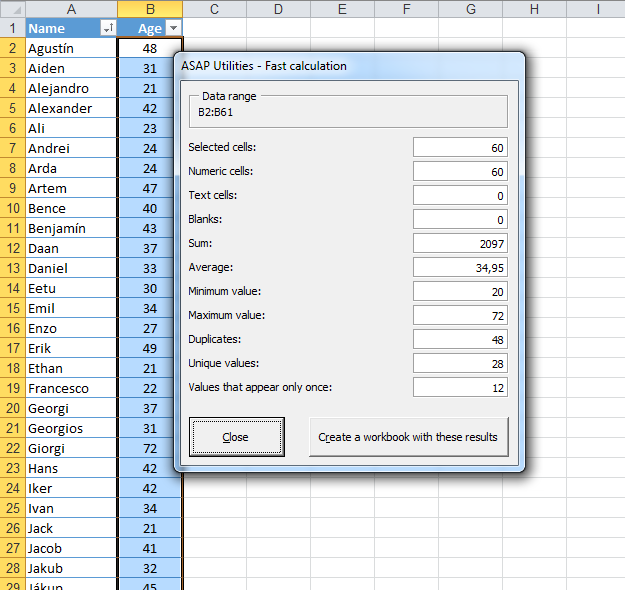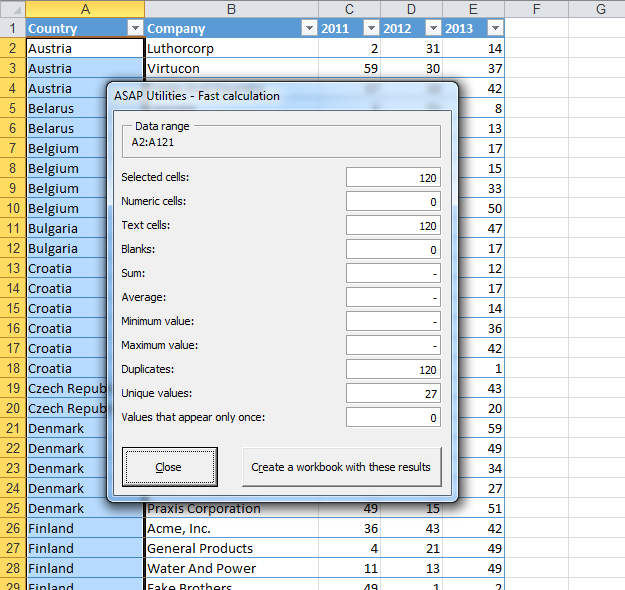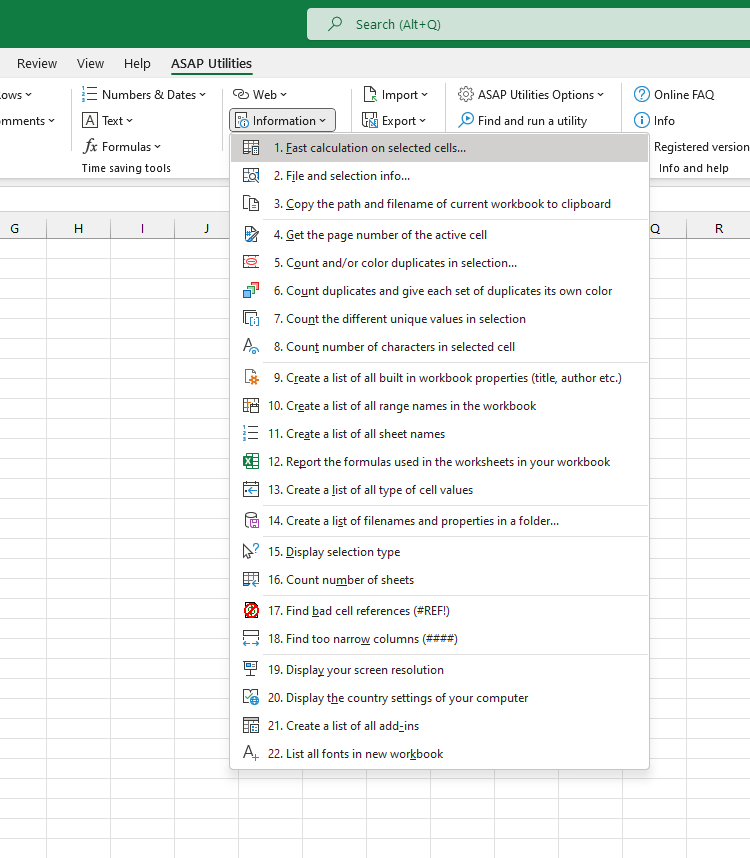# Fast calculation on selected cells...

English (us)NederlandsDeutschEspañolFrançaisPortuguês do BrasilItalianoРусский中文(简体)日本語

## Information  ›  1. Fast calculation on selected cells...

This utility performs a fast calculation on the selected data range.

The utility shows you the following information:
• the number of selected cells
• the number of numeric cells
• the number of text cells
• the number of blank cells
• the sum
• the average
• the minimum value
• the maximum values
• the number of duplicates (values that appear more than once)
• the number of unique values
• values that appear only once

You can choose to have a new workbook created with these results.

Note:
The following rules apply for counting the duplicates and unique values:
The utility is case sensitive which means for example that "Excel" and "EXCEL" is not the same.
If your selection contains formulas then this utility compares the calculated values. This means that this utility considers a cell with the value 12 and a cell with the formula =3*4 as a duplicate.

## Screenshots

Example screenshot: 1Example screenshot: 2